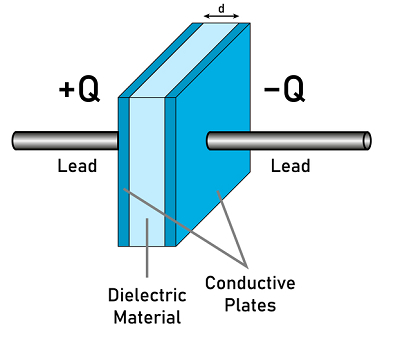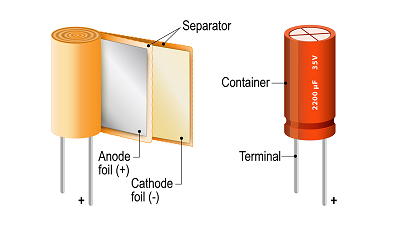# Effect Dielectric Capacitance

## Introduction

Every matter in the universe is made up of molecules. So, many atoms contain both positively charged and negatively charged. This force of attraction is known as a chemical bond. This bonding occurs due to electrostatic force between oppositely charged ions or by sharing of electrons. Formerly called ionic bond and later called a covalent bond. The polarity of the molecules depends upon the bonding between them.

The movement of the charged particles is responsible for electric energy. Energy obtained from the kinetic energy or potential energy of the charged particles is the electric energy. Charged particles are atoms, molecules, or ions that have a charge on them. There is a force of attraction or repulsion between the charged particles depends on the coulomb’s law.

## What is Dielectric?Dielectric materials are bad conductors or insulators. They do not have any free electrons. They are bound with the atom. Free electrons are responsible for the flow of current. As conductors have free electrons they can conduct electricity. Insulators do not have free electrons and so they cannot conduct electricity. As dielectric materials are insulators they do not conduct electricity but they get polarised when an electric field is applied to them. This is known as dielectric polarisation. Paper, glass, mica, and distilled water are some examples of dielectric materials. A vacuum is also an example of dielectric material. Dielectric materials are classified into two as polar dielectric and nonpolar dielectric materials.

## What is Capacitance?

The capability of the materials to store electric charges is called capacitance. Generally, capacitors are made up of two conducting plates which are separated by an insulating material like air, paper, or rubber.

The insulating material that is placed between the conducting plates is called dielectric. When two conducting plates are connected to the battery by connecting one plate to the positive terminal and another one to the negative terminal the electric field makes the electrons move towards the positive terminal. Two conductors are charged, one is positively charged and another one is negatively charged. The dielectric material keeps the electron without crossing the plates.

Thus it stores the energy. This stored energy is utilized when it is connected with any other circuit. Capacitance is the ratio between the charge stored in a conductor and the potential difference.

$$\mathrm{C=\frac{Q}{v}}$$

The S.I unit of capacitance is Farad. The capacitance of the two-plate capacitor is also expressed as,

$$\mathrm{C=\frac{\epsilon _{0}\epsilon _{r}A}{d}}$$

C denotes the capacitance of the capacitor.$\mathrm{\epsilon _{0}}$ denotes the permittivity of free space. $\mathrm{\epsilon _{r}}$denotes the relative permittivity. A denotes the area of the cross-section of the material.

D denoted the distance between the plates.

## Need for Dielectric in a Capacitor

The two main reasons that dielectrics are used in capacitors are given below. As the two conducting plates are connected to the battery and the charges are separated such that one plate contains a positive charge and another one carries a negative charge. The force of attraction between oppositely charged particles tries to connect each other. This reduces the gap. Generally, dielectrics are bad conductors or insulators which reduces this tendency. The electric field between the conductors polarises the molecules of the dielectric material. polarisation reduces the electric field strength. It makes the deposition of more charges. If the electric field is increased the insulator breaks down. Dielectrics have a high breakdown.## Effect of Dielectric on Capacitance

The dielectric materials that are used in the capacitors increase the effective area of the capacitance. Thereby it increases the volumetric efficiency of the capacitance. If the dielectric is a polar molecule, they are in a different orientation in the absence of an electric field. If the electric field is applied they are polarised and produce an electric field in the opposite direction. This reduction of the effective electric field is called the dielectric constant k. The capacitance of the capacitor increased by the factor k.

## The Capacitance of a Capacitor with Dielectric

Let us consider two conductor plates placed parallel with a separation distance of d. A be the area of the cross-section of the plates and the medium separating them is air. If a voltage V is applied to the parallel plate capacitor then the electric field in the capacitor be

$$\mathrm{E=\frac{v }{d}...............(1)}$$

According to Gauss’s law, the electric field is given by,

$$\mathrm{E=\frac{\sigma }{\epsilon _{0}}...............(2)}$$

From equation (1) and (2) we get,

$$\mathrm{\frac{v}{d}=\frac{\sigma }{\epsilon _{0}}}$$

$\mathrm{\sigma}$ denotes the surface charge density. It is defined as the charge per unit area

$$\mathrm{\sigma =\frac{Q}{A}}$$

$$\mathrm{\frac{v}{d}=\frac{\sigma }{\epsilon _{0}}=\frac{Q}{\epsilon _{0}A}}$$

$$\mathrm{V=\frac{Qd}{\epsilon _{0}A}}$$

The capacitance of the capacitor is defined as the ratio between the charge and the potential difference.

$$\mathrm{C=\frac{Q}{v}}$$

$$\mathrm{C=\frac{Q}{v\frac{Qd}{\epsilon _{0}A}}}$$

$$\mathrm{C=Q\:x\frac{\epsilon _{0}A}{Qd}}$$

$$\mathrm{C=\frac{\epsilon _{0}A}{d}}$$

This is the capacitance of the capacitor without the dielectric. If the dielectric is added then the capacitance will become $\mathrm{C=\frac{k\epsilon _{0}A}{d}}$

## Use of Capacitor

Capacitors are used in many places. They are listed below.

• They are used in devices where there is a need for quick release of electric charges like camera flashes and computer keyboards.

• High-frequency electromagnetic radiations are generated and detected by capacitors.

• It is used to store electrical energy for a long period.

• An Ignition system of automobile engines uses capacitors to eliminate sparks.

• It is used in the manufacture of oscillators, amplifiers, transmitters, etc.

• It is used to increase or decrease the impedance of the AC circuit.

## Conclusion

In this tutorial, the dielectric and capacitance are discussed in detail. The need for dielectric in capacitors and the effects of dielectric on capacitance are also discussed in detail. The capacitance of a capacitor with dielectric and the uses of capacitors are also discussed.

## FAQs

Q1. What is dielectric strength and its unit?

Ans. The maximum voltage needed to produce an electric breakthrough in the circuit is known as dielectric strength. It is denoted by a volt per unit thickness (v/m). A vacuum has high dielectric strength.

Q2. What is the dielectric constant? What are the factors that affect the dielectric constant?

Ans. Dielectric constant is the ratio between the permittivity of the material to the permittivity of the free space. It is denoted by k.

Dielectric constant $\mathrm{k=\frac{\epsilon }{\epsilon _{0}}}$

The Dielectric constant is affected by temperature, heating effect, frequency, applied voltage, and humidity.

Q3. What are the applications of dielectric materials?

Ans. Generally, dielectric materials are used as energy storage in capacitors. According to the nature of dielectric material, it can be gaseous, liquid, or solid dielectric. Liquid crystal displays employ dielectric. Dielectric resonator oscillators use ceramic dielectric. Dielectric material with high permittivity is used for the enhancement of the semiconductor. Exposable components are coated by using dielectric material.

Q4. What is the classification of capacitors?

Ans. The capacitors are classified into two. They are polarized capacitors and nonpolarized capacitors. Polarized capacitors are used for only one direction such as positive or negative. Non-polarized capacitors are used in filters.

Q5. Give some examples for non-polarized dielectric materials?

Ans. They are generally used in filtering circuits in electronics. The materials used for this are ceramic, paper, polymer film, and mica.

Updated on: 24-Jan-2023

339 Views